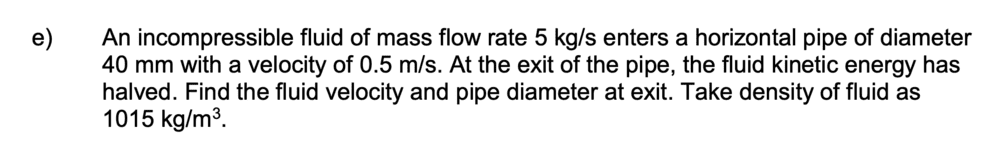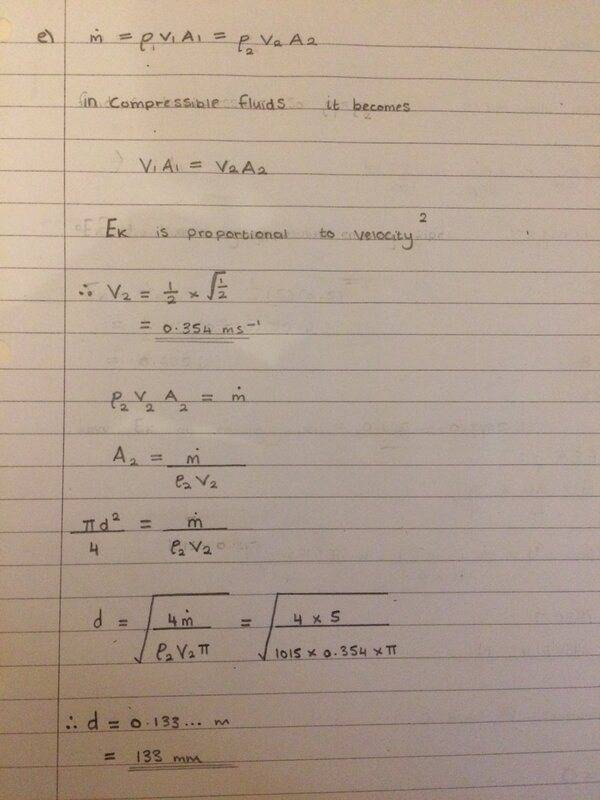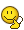# Fluid velocity and pipe diameter using the continuity equation

Bolter
Homework Statement:
See below
Relevant Equations:
continuity equation
Hi,
Can anyone let me know if I had done this Q correctly?Thanks for any help!

•Delta2

Hmm, something about this problem statement seems wrong. If the pipe opening is of diameter ##d_1 = \mathrm{40mm}## and the fluid enters at ##v_1 = \mathrm{0.5ms^{-1}}##, then the mass flow rate should be $$\dot{m} = A_1 \rho v_1 = \frac{\pi}{4} {d_1}^2 \rho v_1 \approx \mathrm{0.64 kg s^{-1}}$$and not ##\mathrm{5 kg s^{-1}}## like they're saying.

You're right to say that if the kinetic energy density halves, then the speed is reduced by a factor of ##\sqrt{2}##, but apart from that the actual question seems inconsistent to me.

Homework Helper
Gold Member
Hmm, something about this problem statement seems wrong. If the pipe opening is of diameter ##d_1 = \mathrm{40mm}## and the fluid enters at ##v_1 = \mathrm{0.5ms^{-1}}##, then the mass flow rate should be $$\dot{m} = A_1 \rho v_1 = \frac{\pi}{4} {d_1}^2 \rho v_1 \approx \mathrm{0.64 kg s^{-1}}$$and not ##\mathrm{5 kg s^{-1}}## like they're saying.

You're right to say that if the kinetic energy density halves, then the speed is reduced by a factor of ##\sqrt{2}##, but apart from that the actual question seems inconsistent to me.
I agree with your numbers but I am disturbed by this problem and I am looking for other ways to interpret what is given and what is being asked. One must take the flow rate and fluid density at face value. I know it's a stretch but one might interpret "enters a horizontal pipe of diameter 40 mm with a velocity of 0.5 m/s" to mean that the fluid has velocity 0.5 m/s before it enters the 40 mm pipe. One then would have to calculate the fluid velocity inside the pipe (your calculation) and use that to find the exit fluid velocity and diameter. Also, there is no mention of pressure in the statement. If one is to use the Bernoulli equation additional assumptions need to be made, e.g. the pressure in the fluid before it enters the 40 mm dia. pipe is atmospheric or some such thing. I don't think this is a well written problem unless there is more to this that we don't know. Note that this is part (e) of a multipart problem.

•Delta2
@kuruman I think this is just a case of a very poorly worded question about the continuity equation for incompressible flow ##\frac{\partial \rho}{\partial t} = -\nabla \cdot \rho\vec{v}##. There will be a pressure change, but I don't think this is important for this question. If we take a surface that coincides with the pipe and its two faces, then $$\frac{dm}{dt} = -\int_{\partial \Omega} \rho \vec{v} \cdot d\vec{S} = 0$$and by extension, since no fluid crosses the pipe walls, then$$\frac{dm}{dt} = -\rho\vec{v}_{\text{in}} \cdot \vec{S}_{\text{inlet}} - \rho \vec{v}_{\text{out}} \cdot \vec{S}_{outlet} = 0$$in the simple case where ##\vec{S}_{\text{inlet}}## and ##\vec{S}_{\text{outlet}}## are parallel, and the flow uniform across a cross section of the fluid, this is more simply described as$$\rho v_{\text{in}} S_{\text{inlet}} = \rho v_{\text{out}} S_{\text{outlet}}$$i.e. we're simply constraining the net flow of mass into the system to be zero.

...but I think the problem-setter has botched the numbersKelvin Perry
I agree with your numbers but I am disturbed by this problem and I am looking for other ways to interpret what is given and what is being asked. One must take the flow rate and fluid density at face value. I know it's a stretch but one might interpret "enters a horizontal pipe of diameter 40 mm with a velocity of 0.5 m/s" to mean that the fluid has velocity 0.5 m/s before it enters the 40 mm pipe. One then would have to calculate the fluid velocity inside the pipe (your calculation) and use that to find the exit fluid velocity and diameter. Also, there is no mention of pressure in the statement. If one is to use the Bernoulli equation additional assumptions need to be made, e.g. the pressure in the fluid before it enters the 40 mm dia. pipe is atmospheric or some such thing. I don't think this is a well written problem unless there is more to this that we don't know. Note that this is part (e) of a multipart problem.
I mistakenly believed that the pressure would be equal to the water's actual pressure, which is 1000 kg/m3, or?

Homework Helper
Gold Member
I mistakenly believed that the pressure would be equal to the water's actual pressure, which is 1000 kg/m3, or?
You also mistakenly believe that the units of pressure are kg/m3. Anyway, this thread is about a year and a half old. Or what?

Homework Helper
Gold Member
2022 Award
"At the exit of the pipe, the fluid kinetic energy has halved."
compared with when? Before entering the pipe or just after?

Staff Emeritus
...but I think the problem-setter has botched the numbers